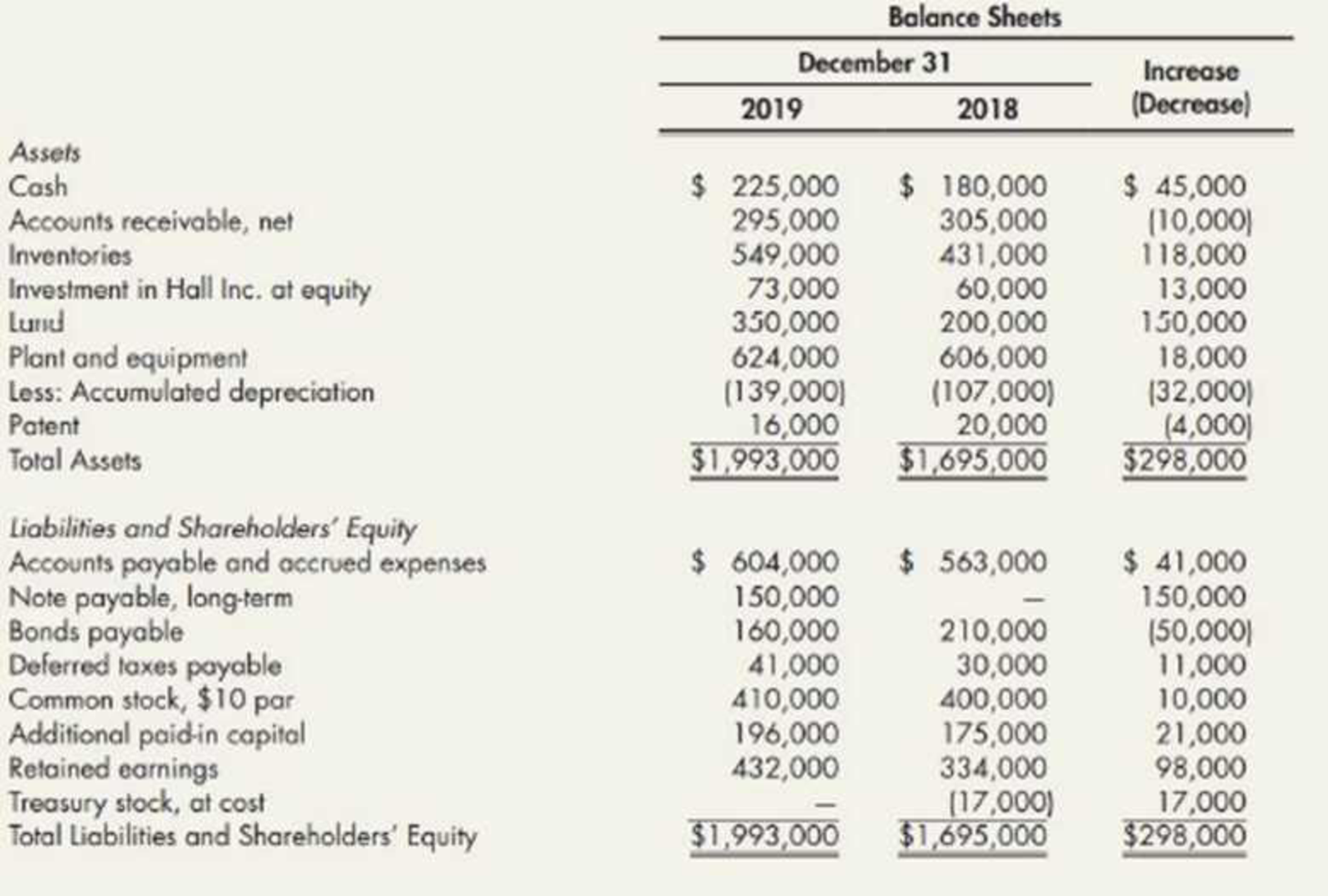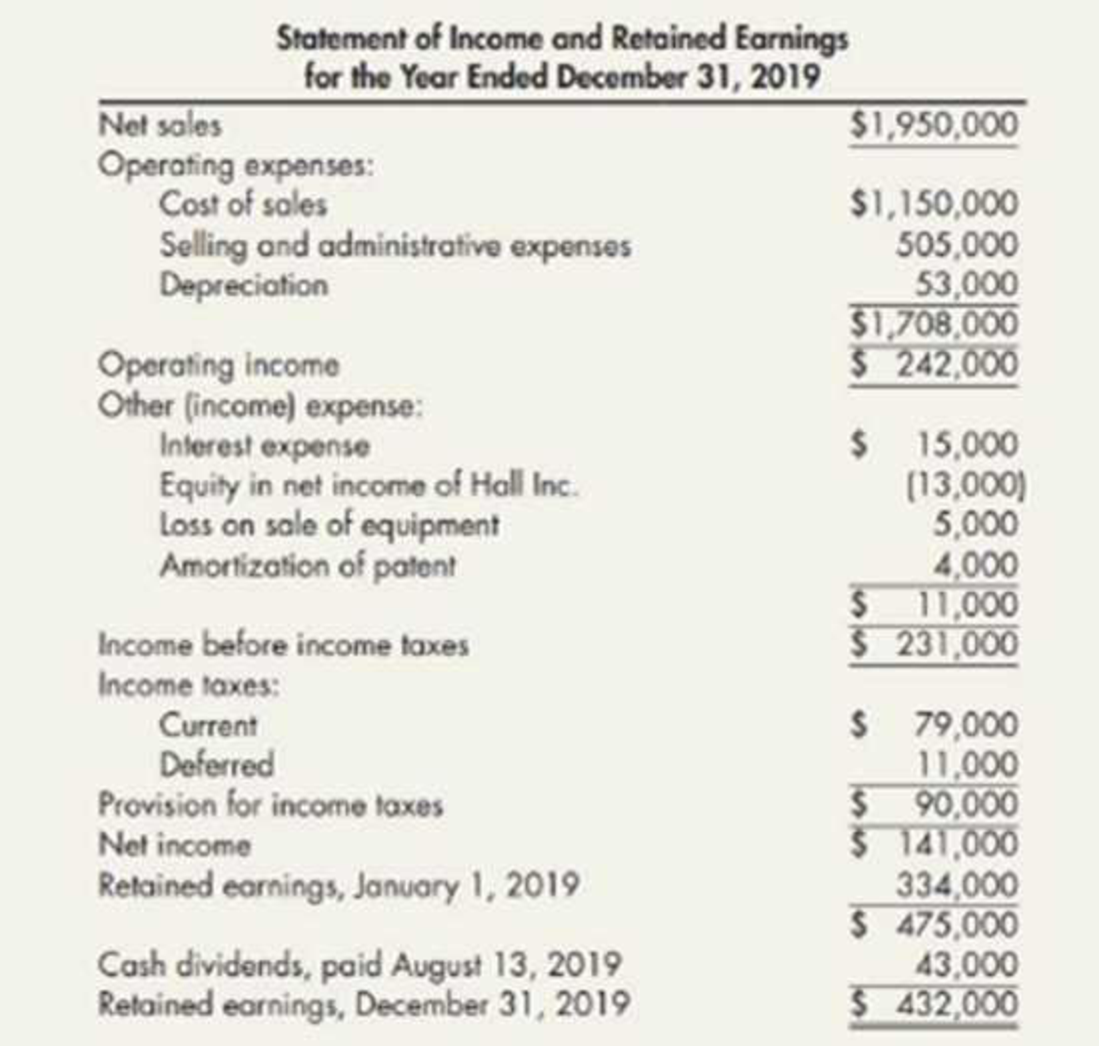Chapter 21, Problem 13P### Intermediate Accounting: Reporting...

3rd Edition
James M. Wahlen + 2 others
ISBN: 9781337788281

#### Solutions

Chapter
Section### Intermediate Accounting: Reporting...

3rd Edition
James M. Wahlen + 2 others
ISBN: 9781337788281
Textbook Problem
51 views

# Comprehensive The following are Farrell Corporation’s balance sheets as of December 31, 2019, and 2018, and the statement of income and retained earnings for the year ended December 31, 2019:Additional information: a. On January 2, 2019, Farrell sold equipment costing $45,000, with a book value of$24,000, for $19,000 cash. b. On April 2, 2019, Farrell issued 1,000 shares of common stock for$23,000 cash. c. On May 14, 2019, Farrell sold all of its treasury stock for $25,000 cash. d. On June 1, 2019, Farrell paid$50,000 to retire bonds with a face value (and book value) of $50,000. e. On July 2, 2019, Farrell purchased equipment for$63,000 cash. f. On December 31, 2019. land with a fair market value of $150,000 was purchased through the issuance of a long-term note in the amount of$150,000. The note bears interest at the rate of 15% and is due on December 31, 2021. g. Deferred taxes payable represent temporary differences relating to the use of accelerated depreciation methods for income tax reporting and the straight-line method for financial statement reporting. Required: 1. Prepare a spreadsheet to support a statement of cash flows for Farrell for the year ended December 31, 2019, based on the preceding information. 2. Prepare the statement of cash flows.

1.

To determine

Prepare worksheet entries to support the cash flow statement of F Company for the year ended December 31, 2019.

Explanation

Worksheet: A worksheet is a spreadsheet used while preparing a financial statement. It is a type of form having multiple columns and it is used in the adjustment process. The use of a worksheet is optional for any organization. A worksheet can neither be considered as a journal nor a part of the general ledger.

Prepare worksheet entries of F Company for the year ended December 31, 2019.

Table (1)

Table (2)

Notes:

a) Net income for the year ended December 31, 2019 is $141,000. b) Amount of depreciation is$53,000.

c) Income from equity share of H Company is $13,000. d) Amortization expense for patent is$4,000.

e) Calculate decrease in accounts payable.

Decrease in accounts receivable = (Beginnig year's accounts receivable – Ending year's accounts receivable) = $305,000$2,95,00=$10,000 f) Calculate increase in inventory Increase in inventory = Closing inventory - opening inventory=$549,000$431,000=$118,000

g) Calculate increase in accounts payable and accrued expense.

Increase in accounts payableand accrued expense) = (Closing balance of accounts payable and accrued expenseOpening balance of accounts payable and accrued expense)=$604,000$563,000=$41,000 h) Calculate loss on sale of equipment. Loss on sale of equipment =(Book value of equipmentSelling price)=$24,000$19,000=$5,000

i) Calculate proceeds from common stock

2.

To determine

Prepare cash flow statement under indirect method of F Company for the year ended 31st December 2019.

### Still sussing out bartleby?

Check out a sample textbook solution.

See a sample solution

#### The Solution to Your Study Problems

Bartleby provides explanations to thousands of textbook problems written by our experts, many with advanced degrees!

Get Started